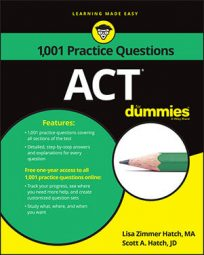##### ACTIt's nice to be color-coordinated and even physically coordinated, but, on the ACT Math exam, it's really useful to be skilled at coordinate geometry. To help you prepare, the following practice questions ask you to find a line that is perpendicular to a given line, and then find the distance between two given points.

## Practice questions

Use the following graph to solve the first practice question.

1. What would the slope be of any line in the standard xy-coordinate plane that is perpendicular to the line l in the graph?

2. What is the distance in the standard xy-coordinate plane between the points (–2, 1) and (3, –2)?

1. The correct answer is Choice (A).

The slope of a perpendicular line is the opposite reciprocal of the slope of the line it intersects. The figure shows line l with a positive slope, so the slope of a perpendicular line has to be negative. Eliminate Choices (D) and (E).

To choose among the remaining answers, find the slope of the given line. The graph shows that the given line travels through the points (–5, 2) and (7, 3). Plug values into the slope equation to solve:

Switch the sign to negative and flip the numerator and denominator. The slope is –12.

2. The correct answer is Choice (C).

You can solve this question is by plugging the given values into the distance formula and solving: